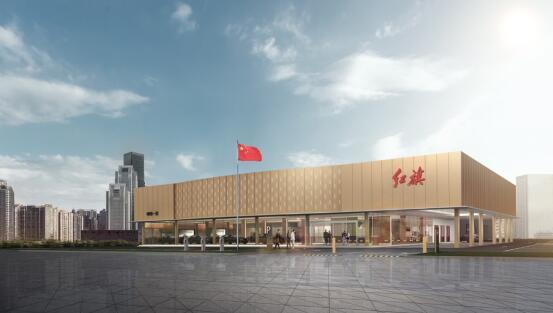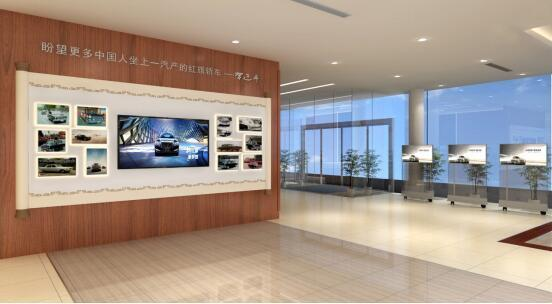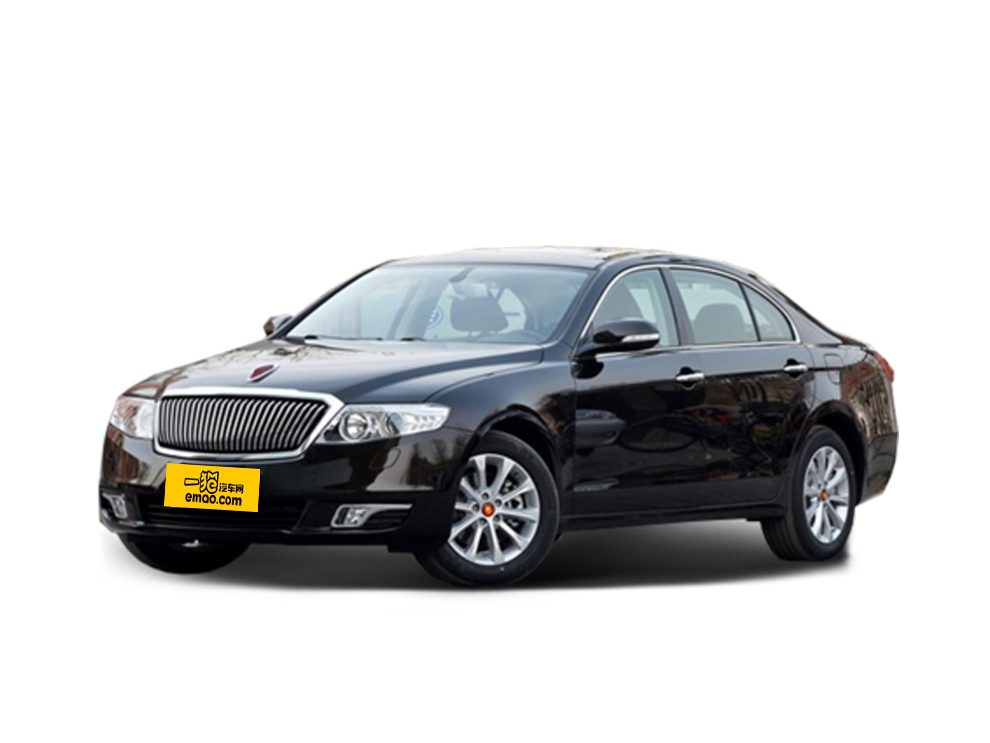经过多年来的不断变革，我国的汽车行业渠道建设得到了较快发展，由单一化逐渐演变为多元化，每一个市场阶段的需求都在引导着汽车行业渠道建设的变革。作为中国豪华车第一品牌，红旗正紧随时代步伐，将目光聚焦于渠道建设创新之中。近日，红旗品牌的营销团队，经长春、郑州、杭州、成都、广州五地，圆满完成了红旗经销商全国巡回座谈会，加速推进了红旗品牌渠道建设的发展。正所谓“一分耕耘，一分收获”。根据规划，红旗品牌将于2018年3月实现全国100家经销商的覆盖。如今，红旗全员上下一心，明年完成覆盖100家经销商的“小目标”已毫无难度，但红旗并不满足于现状，不仅会紧抓预期目标，同时将打破传统渠道建设的束缚，从更加直接的用户体验角度出发，打造一个前所未有的“红旗体验中心”。习总书记曾提出“盼望更多中国人坐上一汽产的红旗轿车”，红旗也正是在这样的号召下，在品牌战略和渠道发展策略方面做出了重大调整。全新的红旗体验中心将为客户带来超越普通4S店的销售标准与服务质量，重点围绕客户体验展开设计和建设，为客户带来更加愉悦的全方位体验。在红旗体验中心，客户除了能够感受更高标准的产品体验、销售体验和服务体验外，还将亲身体验到“国车之魂”厚重且独特的民族情怀。客户在整个体验过程中，也能够充分了解到红旗品牌的悠久历史、品牌精神，感受独具东方魅力的豪华体验。
红旗不仅在渠道建设上进行了创新，在渠道布局层面也同样令人刮目相看。红旗向世界展现“东方豪华”的独特魅力，将招商范围突破性的布局到了澳门特别行政区，让红旗品牌深厚的民族文化传承与大国科技的精髓走向前沿，成为中国与世界汽车文化沟通交流的纽带。
作为中国最重要的国际化都市之一，澳门是中国与世界经济、文化交流的“前沿阵地”。红旗体验中心落户澳门，将进一步提高其国际曝光率和市场影响力，为红旗成为世界一流汽车品牌奠定坚实基础。同时，今年作为澳门回归祖国的18周年，此次红旗将在澳门落子，既是对澳门回归18周年的献礼，也将成为推动中国汽车品牌融入世界汽车领域的一次盛举。
红旗在渠道建设上的创新，将为用户带来焕然一新的极致体验，也与品牌发展形成深度共鸣。红旗体验中心将为用户打造一个与生活息息相关的连接点，为用户提供更加丰富的特色文化体验活动，让用户能够以红旗体验中心为生活据点，为个人创享生活带来更多新选择。
一面是经销网络扩建，一面是打造全新用户体验，红旗这一系列突破性举措，将使红旗以极具东方魅力的面貌与广大用户见面，也将进一步巩固其中国豪华车领导者地位。目前，红旗品牌的渠道建设已初见成效，其良好的发展态势更值得国人期待。
历经近60年风风雨雨，红旗品牌早已成为了国人心中的一面旗帜，凝结着新中国汽车工业的结晶和中国汽车工业的希望。红旗品牌背负着国人的太多情感和责任，它代表的是中国汽车的形象。我们期待能够在有温度、有传承的红旗体验中心里，唤醒每一位国人心中的民族情怀，让“红旗之梦”触手可及。

##### 相关资讯

|分享

•已阅！握爪
•我手滑为你点赞
•128个赞！
•不明觉厉
•阅后既醉
•有钱！任性
•照片太美，我不敢看
•干货！杠杠的
•高大上
•膜拜中！
•请接受我的膝盖
•猫哥/猫妹么么哒
•神吐槽
•我只笑笑不说话
•我想静静
•窒息
##### 资讯相关车系##### 热度排行• 阿斯顿·马丁
• 奥迪
• 阿尔法罗密欧
• ALPINA

• 巴博斯
• 宝骏
• 宝马
• 保时捷
• 北汽制造
• 奔驰
• 奔腾
• 本田
• 比亚迪
• 标致
• 别克
• 宾利
• 布加迪
• 北汽威旺
• 北京
• 北汽绅宝
• 北汽幻速
• 北汽新能源
• 宝沃
• 比速汽车
• 北汽道达

• 昌河
• 长安
• 长城
• 长安商用
• 成功汽车
• 长江EV
• 长安轻车型

• 大众
• 道奇
• 东风
• 东风风神
• 东风小康
• 东南
• DS
• 东风风行
• 东风风度
• 东风风光

• 法拉利
• 菲亚特
• 丰田
• 福迪
• 福特
• 福田汽车
• 福汽启腾
• 风诺

• GMC
• 广汽传祺
• 广汽吉奥
• 观致

• 哈飞
• 海格
• 海马
• 华泰
• 黄海
• 恒天
• 红旗
• 哈弗
• 华颂
• 华凯
• 华泰新能源
• 汉腾汽车

• Jeep
• 江淮
• 江铃
• 捷豹
• 金杯
• 九龙
• 吉利汽车
• 金旅
• 金龙
• 江铃集团轻汽
• 江铃集团新能源
• 君马汽车
• 捷途

• 开瑞
• 凯迪拉克
• 科尼赛克
• 克莱斯勒
• KTM
• 卡威
• 凯翼
• 康迪
• 康迪电动汽车集团

• 猎豹汽车
• 兰博基尼
• 劳斯莱斯
• 雷克萨斯
• 雷诺
• 理念
• 力帆
• 莲花汽车
• 林肯
• 铃木
• 陆风
• 路虎
• 路特斯
• 领志
• 领克
• 零跑汽车

• MG
• MINI
• 马自达
• 玛莎拉蒂
• 迈凯伦
• 摩根

• 纳智捷

• 讴歌
• 欧宝
• 欧朗
• 欧拉
• 欧尚汽车

• 帕加尼

• 奇瑞
• 启辰
• 起亚
• 前途
• 庆铃汽车

• 日产
• 荣威
• 瑞麒汽车
• 如虎
• 瑞驰

• 上汽大通
• smart
• 三菱
• 双环
• 双龙
• 斯巴鲁
• 斯柯达
• 萨博
• 思铭
• 赛麟
• SWM斯威汽车

• TESLA
• 泰卡特
• 腾势

• 威麟
• 威兹曼
• 沃尔沃
• 五菱汽车
• 五十铃
• 潍柴英致
• WEY
• 蔚来
• 威马汽车

• 现代
• 雪佛兰
• 雪铁龙
• 西雅特
• 新特汽车
• 小鹏汽车
• 新宝骏

• 野马汽车
• 一汽
• 依维柯
• 英菲尼迪
• 永源
• 驭胜

• 中华
• 中兴
• 众泰
• 知豆
• 之诺
• 正道汽车
• A
• B
• C
• D
• E
• F
• G
• H
• I
• J
• K
• L
• M
• N
• O
• P
• Q
• R
• S
• T
• U
• V
• W
• X
• Y
• Z

• 阿斯顿·马丁
• 奥迪
• 阿尔法罗密欧
• ALPINA

• 巴博斯
• 宝骏
• 宝马
• 保时捷
• 北汽制造
• 奔驰
• 奔腾
• 本田
• 比亚迪
• 标致
• 别克
• 宾利
• 布加迪
• 北汽威旺
• 北京
• 北汽绅宝
• 北汽幻速
• 北汽新能源
• 宝沃
• 比速汽车
• 北汽道达

• 昌河
• 长安
• 长城
• 长安商用
• 成功汽车
• 长江EV
• 长安轻车型

• 大众
• 道奇
• 东风
• 东风风神
• 东风小康
• 东南
• DS
• 东风风行
• 东风风度
• 东风风光

• 法拉利
• 菲亚特
• 丰田
• 福迪
• 福特
• 福田汽车
• 福汽启腾
• 风诺

• GMC
• 广汽传祺
• 广汽吉奥
• 观致

• 哈飞
• 海格
• 海马
• 华泰
• 黄海
• 恒天
• 红旗
• 哈弗
• 华颂
• 华凯
• 华泰新能源
• 汉腾汽车

• Jeep
• 江淮
• 江铃
• 捷豹
• 金杯
• 九龙
• 吉利汽车
• 金旅
• 金龙
• 江铃集团轻汽
• 江铃集团新能源
• 君马汽车
• 捷途

• 开瑞
• 凯迪拉克
• 科尼赛克
• 克莱斯勒
• KTM
• 卡威
• 凯翼
• 康迪
• 康迪电动汽车集团

• 猎豹汽车
• 兰博基尼
• 劳斯莱斯
• 雷克萨斯
• 雷诺
• 理念
• 力帆
• 莲花汽车
• 林肯
• 铃木
• 陆风
• 路虎
• 路特斯
• 领志
• 领克
• 零跑汽车

• MG
• MINI
• 马自达
• 玛莎拉蒂
• 迈凯伦
• 摩根

• 纳智捷

• 讴歌
• 欧宝
• 欧朗
• 欧拉
• 欧尚汽车

• 帕加尼

• 奇瑞
• 启辰
• 起亚
• 前途
• 庆铃汽车

• 日产
• 荣威
• 瑞麒汽车
• 如虎
• 瑞驰

• 上汽大通
• smart
• 三菱
• 双环
• 双龙
• 斯巴鲁
• 斯柯达
• 萨博
• 思铭
• 赛麟
• SWM斯威汽车

• TESLA
• 泰卡特
• 腾势

• 威麟
• 威兹曼
• 沃尔沃
• 五菱汽车
• 五十铃
• 潍柴英致
• WEY
• 蔚来
• 威马汽车

• 现代
• 雪佛兰
• 雪铁龙
• 西雅特
• 新特汽车
• 小鹏汽车
• 新宝骏

• 野马汽车
• 一汽
• 依维柯
• 英菲尼迪
• 永源
• 驭胜

• 中华
• 中兴
• 众泰
• 知豆
• 之诺
• 正道汽车
• A
• B
• C
• D
• E
• F
• G
• H
• I
• J
• K
• L
• M
• N
• O
• P
• Q
• R
• S
• T
• U
• V
• W
• X
• Y
• Z

• 阿斯顿·马丁
• 奥迪
• 阿尔法罗密欧
• ALPINA

• 巴博斯
• 宝骏
• 宝马
• 保时捷
• 北汽制造
• 奔驰
• 奔腾
• 本田
• 比亚迪
• 标致
• 别克
• 宾利
• 布加迪
• 北汽威旺
• 北京
• 北汽绅宝
• 北汽幻速
• 北汽新能源
• 宝沃
• 比速汽车
• 北汽道达

• 昌河
• 长安
• 长城
• 长安商用
• 成功汽车
• 长江EV
• 长安轻车型

• 大众
• 道奇
• 东风
• 东风风神
• 东风小康
• 东南
• DS
• 东风风行
• 东风风度
• 东风风光

• 法拉利
• 菲亚特
• 丰田
• 福迪
• 福特
• 福田汽车
• 福汽启腾
• 风诺

• GMC
• 广汽传祺
• 广汽吉奥
• 观致

• 哈飞
• 海格
• 海马
• 华泰
• 黄海
• 恒天
• 红旗
• 哈弗
• 华颂
• 华凯
• 华泰新能源
• 汉腾汽车

• Jeep
• 江淮
• 江铃
• 捷豹
• 金杯
• 九龙
• 吉利汽车
• 金旅
• 金龙
• 江铃集团轻汽
• 江铃集团新能源
• 君马汽车
• 捷途

• 开瑞
• 凯迪拉克
• 科尼赛克
• 克莱斯勒
• KTM
• 卡威
• 凯翼
• 康迪
• 康迪电动汽车集团

• 猎豹汽车
• 兰博基尼
• 劳斯莱斯
• 雷克萨斯
• 雷诺
• 理念
• 力帆
• 莲花汽车
• 林肯
• 铃木
• 陆风
• 路虎
• 路特斯
• 领志
• 领克
• 零跑汽车

• MG
• MINI
• 马自达
• 玛莎拉蒂
• 迈凯伦
• 摩根

• 纳智捷

• 讴歌
• 欧宝
• 欧朗
• 欧拉
• 欧尚汽车

• 帕加尼

• 奇瑞
• 启辰
• 起亚
• 前途
• 庆铃汽车

• 日产
• 荣威
• 瑞麒汽车
• 如虎
• 瑞驰

• 上汽大通
• smart
• 三菱
• 双环
• 双龙
• 斯巴鲁
• 斯柯达
• 萨博
• 思铭
• 赛麟
• SWM斯威汽车

• TESLA
• 泰卡特
• 腾势

• 威麟
• 威兹曼
• 沃尔沃
• 五菱汽车
• 五十铃
• 潍柴英致
• WEY
• 蔚来
• 威马汽车

• 现代
• 雪佛兰
• 雪铁龙
• 西雅特
• 新特汽车
• 小鹏汽车
• 新宝骏

• 野马汽车
• 一汽
• 依维柯
• 英菲尼迪
• 永源
• 驭胜

• 中华
• 中兴
• 众泰
• 知豆
• 之诺
• 正道汽车
• A
• B
• C
• D
• E
• F
• G
• H
• I
• J
• K
• L
• M
• N
• O
• P
• Q
• R
• S
• T
• U
• V
• W
• X
• Y
• Z